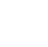# Java Variables

A variable is a name given to a memory location. It is the basic unit of storage in a program. The value stored in a variable can be changed during program execution. In Java, all the variables must be declared before use.

## 1. Declaring variables

• datatype: Type of data that can be stored in this variable.
• variable_name: Name given to the variable.

Syntax:

``` data_type variable_name; ```

Example:

``` char alphabet ; int number; ```

Where alphabet and number  are then variables  of type character and integer.

## 2. Assigning values to variables

Values can be assigned to a variable directly using assignment operator(=).

Syntax:

``` data_type variable_name = value; ```

Example:

``` char alphabet = 'A'; int number = 100; ```

Where A and 100 are the values assigned to the variable alphabet and number.

## 3. Rules for Naming Variables

• Variable names may consists of alphabets , digits , underscore ( _ ) and dollar sign ( \$ ).
• They must not be begin with digits.
• It should not be a keyword.
• White space is not allowed.
• Variable names can't be in length.
• Uppercase and Lowercase are distinct.

## Valid and Invalid Variable Names :

``` int sum_value = 10; // ✔️ valid ( underscores are allowed ) int \$value = 20;    // ✔️ valid ( dollor sign is allowed ) int 12sum = 10;     // ❌ invalid ( variable name can not start with digits ) int break = 20;     // ❌ invalid ( variable names should not be a keyword ) int sum value = 30; // ❌ invalid ( whitespace is not allowed ) ```